# User Manual: High-Dimensional Conditional Average Treatment Effects Estimation (R Package)

This package uses a two-step procedure to estimate the conditional average treatment effects (CATE) with potentially high-dimensional covariate(s). In the first stage, the nuisance functions necessary for identifying CATE can be estimated by machine learning methods, allowing the number of covariates to be comparable to or larger than the sample size. The second stage consists of a low-dimensional local linear regression, reducing CATE to a function of the covariate(s) of interest.

The CATE estimator implemented in this package not only allows for high-dimensional data, but also has the “double robustness” property: either the model for the propensity score or the models for the conditional means of the potential outcomes are allowed to be misspecified (but not both).

The algorithm used in this package is described in the paper by Fan et al., “Estimation of Conditional Average Treatment Effects With High-Dimensional Data” (2022), Journal of Business & Economic Statistics. (doi:10.1080/07350015.2020.1811102)

## Installation

Install the package from github:

install.packages('devtools')
devtools::install_github('microfan1/hdcate')

## Basic Usage

library(hdcate)

Define the empirical data (which is a usual data.frame), here we use a built-in simulated data:

set.seed(1)  # set an arbitrary random seed to ensure the reproducibility
data <- HDCATE.get_sim_data(n_obs=500, n_var=100, n_rel_var=4, intercept=10)  # generate data
#> Actual CATE function is: CATE(x)=10+1*x

The simulated data contains 500 observations, each observation has 100 covariates, only the first 4 of those covariates (X1, X2, X3 and X4) are actually present in the expectation function of potential outcome, and only the last 4 covariates (X97, X98, X99 and X100) are present in the propensity score function.

Let’s see what the data looks like:

data
#>             Y D           X1           X2           X3           X4
#> 1    0.000000 0 -0.626453811  0.077303123  1.134965089  0.850043472
#> 2    0.000000 0  0.183643324 -0.296868642  1.111931845 -0.925312995
#> 3    0.000000 0 -0.835628612 -1.183242240 -0.870777634  0.893581214
#> 4    0.000000 0  1.595280802  0.011292688  0.210731585 -0.941009738
#> 5    0.000000 0  0.329507772  0.991601036  0.069395647  0.538952094
....

In the data, we know that the dependent variable is Y, treatment variable is D, and we use a model with the formula of independent variable as:

x_formula <- "X1+X2+X3+X4+X5+X6"
x_formula
#>  "X1+X2+X3+X4+X5+X6"

Note that the propensity score function is misspecified in this example. We shall see the “double robust” shortly.

Then, we can use the data to create a model:

model <- HDCATE(data=data, y_name='Y', d_name='D', x_formula=x_formula)

Pass this model to an operating function HDCATE.set_condition_var() to set the required conditional variable, here we want to know the conditional average treatment effects (CATE) for X2, from X2=-1 to X2=1, step by 0.01:

HDCATE.set_condition_var(model, 'X2', min=-1, max=1, step=0.01)

Then we are ready to fit the model, pass the model to the operating function HDCATE.fit() to fit the model:

HDCATE.fit(model)

After the model fitting, we obtain the resulting average treatment effects (via accesing the ate attribute of model) and conditional average treatment effects (via accesing the hdcate attribute of model):

# ATE
model$ate #>  9.874983 # CATE model$hdcate
#>     8.989368  8.994602  9.001866  9.011095  9.022186  9.035010  9.049411
#>     9.065215  9.082236  9.100283  9.119161  9.138682  9.158664  9.178935
#>    9.199339  9.219731  9.239988  9.260001  9.279679  9.298949  9.317753
#>    9.336050  9.353811  9.371020  9.387670  9.403765  9.419310  9.434320
#>    9.448809  9.462793  9.476288  9.489310  9.501875  9.513993  9.525677
#>    9.536936  9.547775  9.558199  9.568210  9.577807  9.586984  9.595733
....

After fitting, we may want to know the uniform confidence bands, to achieve this, pass the fitted model to the operating function HDCATE.inference():

HDCATE.inference(model)

Then we obtain the uniform confidence bands for 2-sided test, left-sided test and right-sided test by accessing the i_two_side, i_left_side and i_right_side attributes of the model, respectively:

# two-sided bands
model$i_two_side #> Sigificant level 0.01 Sigificant level 0.01 #> CATE on X2=-1 8.070107 9.908629 #> CATE on X2=-0.99 8.079787 9.909418 #> CATE on X2=-0.98 8.091711 9.912022 #> CATE on X2=-0.97 8.105821 9.916368 #> CATE on X2=-0.96 8.122017 9.922356 .... # left-sided bands model$i_left_side
#>                                Sigificant level 0.01
#> CATE on X2=-1                               8.070107
#> CATE on X2=-0.99                            8.079787
#> CATE on X2=-0.98                            8.091711
#> CATE on X2=-0.97                            8.105821
#> CATE on X2=-0.96                            8.122017
....
# right-sided bands
model$i_right_side #> Sigificant level 0.01 #> CATE on X2=-1 9.908629 #> CATE on X2=-0.99 9.909418 #> CATE on X2=-0.98 9.912022 #> CATE on X2=-0.97 9.916368 #> CATE on X2=-0.96 9.922356 .... Finally, we may want a plot for the estimated results above: HDCATE.plot(model) # Alternatively, if the model is not inferenced, use the code below: # HDCATE.plot(model, include_band=FALSE) # Alternatively, we may want to save the full plot as a high-definition PDF file: # HDCATE.plot(model, output_pdf=TRUE, pdf_name='hdcate_plot.pdf', # include_band=TRUE, test_side='both', y_axis_min='auto', y_axis_max='auto', # display.hdcate='HDCATEF', display.ate='ATE', display.siglevel='sig_level' # )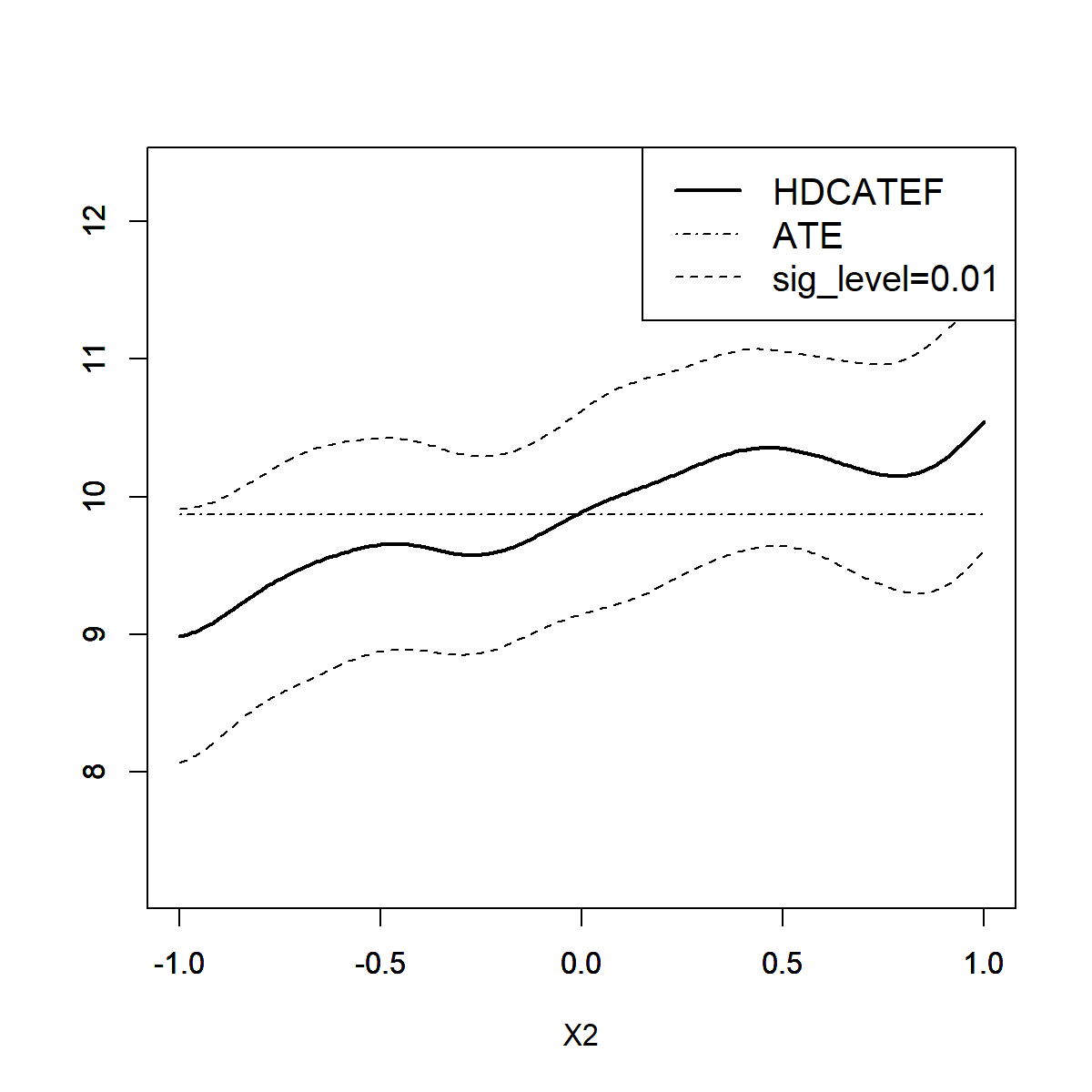HDCATE Estimation (propensity function is misspecified) From the graph above, although the model we use is misspecified for the propensity score function (we used the formula Y~X1+X2+X3+X4+X5+X6), we still obtain a robust result, which is very close to the actual CATE function (CATE(X2)=10+X2). If we use a model that is misspecified for the expectation function of potential outcome, the results are also robust: x_formula = paste(paste0('X', c(90:100)), collapse ='+') # x_formula="X90+...+X100" model <- HDCATE(data=data, y_name='Y', d_name='D', x_formula=x_formula) HDCATE.set_condition_var(model, 'X2', min=-1, max=1, step=0.01) HDCATE.fit(model) HDCATE.inference(model) HDCATE.plot(model)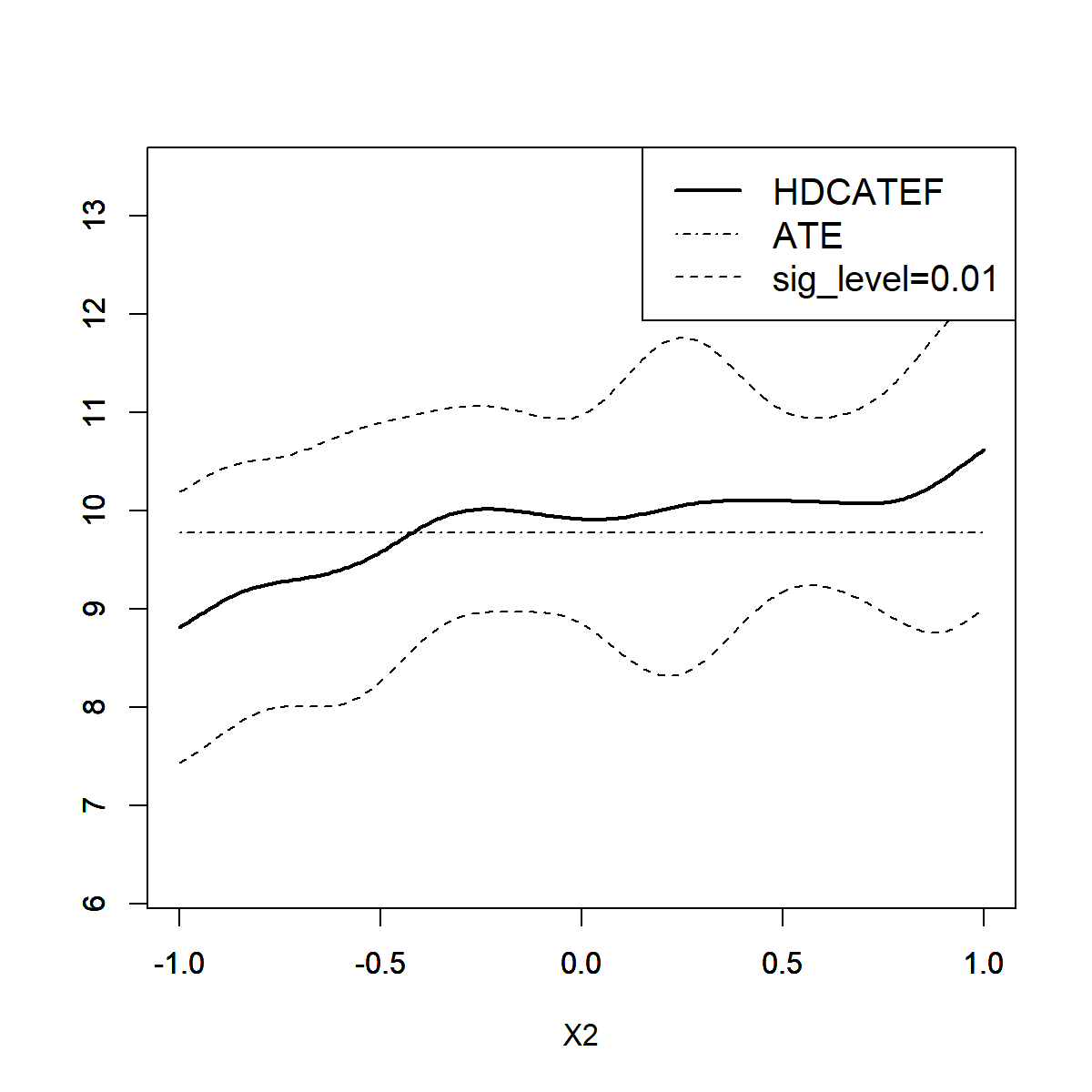HDCATE Estimation (expectation function is misspecified) The results above show the “double robust” property of the estimating approach in this package, see Fan et al. (2022) for more theoretical results. ## Advanced Usage ### Full-sample Estimator vs Cross-fitting Estimator By default, when creating an HDCATE model, this package uses full-sample approach, but you are also very welcome to use the cross-fitting approach described in Fan et al. (2022), by simply pass model into the operator HDCATE.use_cross_fitting() right after the HDCATE model has been created, that is: # create the model first, or use an existing model and skip this line model <- HDCATE(data=data, y_name='Y', d_name='D', x_formula=x_formula) # suppose we want to use the 5-fold cross-fitting approach: HDCATE.use_cross_fitting(model, k_fold=5) You may also want to set the folds manually, in this case, pass a list of index vector to the third argument of the operator: # In this case, the param k_fold is auto detected, you can pass any value to it. HDCATE.use_cross_fitting(model, k_fold=1, folds=list(c(1:250), c(251:500))) If we wants to use full-sample approach again, then we can use anothor operator HDCATE.use_full_sample(). HDCATE.use_full_sample(model) ### User-defined Machine Learning Approach By default, the package uses LASSO on the first stage (i.e. the estimation of the treated/untreated expectation functions and propensity score functions), but it is highly (and easily) extendable. Researchers are welcome to define their own preferred methods (such as other ML methods) to run the first-stage estimation. To define your own approach, you should define several functions that describe how you fit and predict the input data, and then pass those functions (and the model object) to the operator HDCATE.set_first_stage(). Then the package will use your method on the first stage. The template of those functions are given as follows: my_method_to_fit_expectation <- function(df) { # fit the input data.frame (df), where the first column is outcome value (Y) # and the rest are covariates in x_formula # ... # return a fitted object } my_method_to_predict_expectation <- function(fitted_model, df) { # use the fitted object (returned by your function above) to predict the conditional expectation # fucntion on the input data.frame (df) # ... # return a vector of predicted values } my_method_to_fit_propensity <- function(df) { # fit the input data.frame (df), where the first column is dummy treatment # value (D) and the rest are covariates in x_formula # ... # return a fitted object } my_method_to_predict_propensity <- function(fitted_model, df) { # use the fitted object (returned by your function above) to predict the propensity score # fucntion on the input data.frame (df) # ... # return a vector of predicted values } Example 1 (LASSO): the default ML method in HDCATE.fit is LASSO, users can manually define a ML method using the above template, and we again use LASSO as an example, similar practice applies for other ML methods: Note: In practice, LASSO is the built-in method in the package, if you just want to use LASSO, there’s no need to do the following. x_formula <- "X1+X2+X3+X4+X5+X6" # propensity function is misspecified # create a model model <- HDCATE(data=data, y_name='Y', d_name='D', x_formula=x_formula) # using the template above, we can write: my_method_to_fit_expectation <- function(df) { # fit the input data.frame (df), where the first column is outcome value (Y) # and the rest are covariates in x_formula hdm::rlasso(as.formula(paste0('Y', "~", x_formula)), df) # return a fitted object } my_method_to_predict_expectation <- function(fitted_model, df) { # use the fitted object (fitted_model) to predict the conditional expectation # fucntion on the input data.frame (df) predict(fitted_model, df) # return a vector of predicted values } my_method_to_fit_propensity <- function(df) { # fit the input data.frame (df), where the first column is dummy treatment # variable (D) and the rest are covariates in x_formula hdm::rlassologit(as.formula(paste0('D', "~", x_formula)), df) # return a fitted object } my_method_to_predict_propensity <- function(fitted_model, df) { # use the fitted object (fitted_model) to predict the propensity score # fucntion on the input data.frame (df) predict(fitted_model, df, type="response") # return a vector of predicted values } After defining these functions, we can easily plug them into the package using operator HDCATE.set_first_stage(): HDCATE.set_first_stage( model, fit.treated=my_method_to_fit_expectation, fit.untreated=my_method_to_fit_expectation, fit.propensity=my_method_to_fit_propensity, predict.treated=my_method_to_predict_expectation, predict.untreated=my_method_to_predict_expectation, predict.propensity=my_method_to_predict_propensity ) And then we can use the user-defined first-stage ML methods to estimate CATE: HDCATE.set_condition_var(model, 'X2', min=-1, max=1, step=0.01) HDCATE.fit(model) HDCATE.inference(model) HDCATE.plot(model)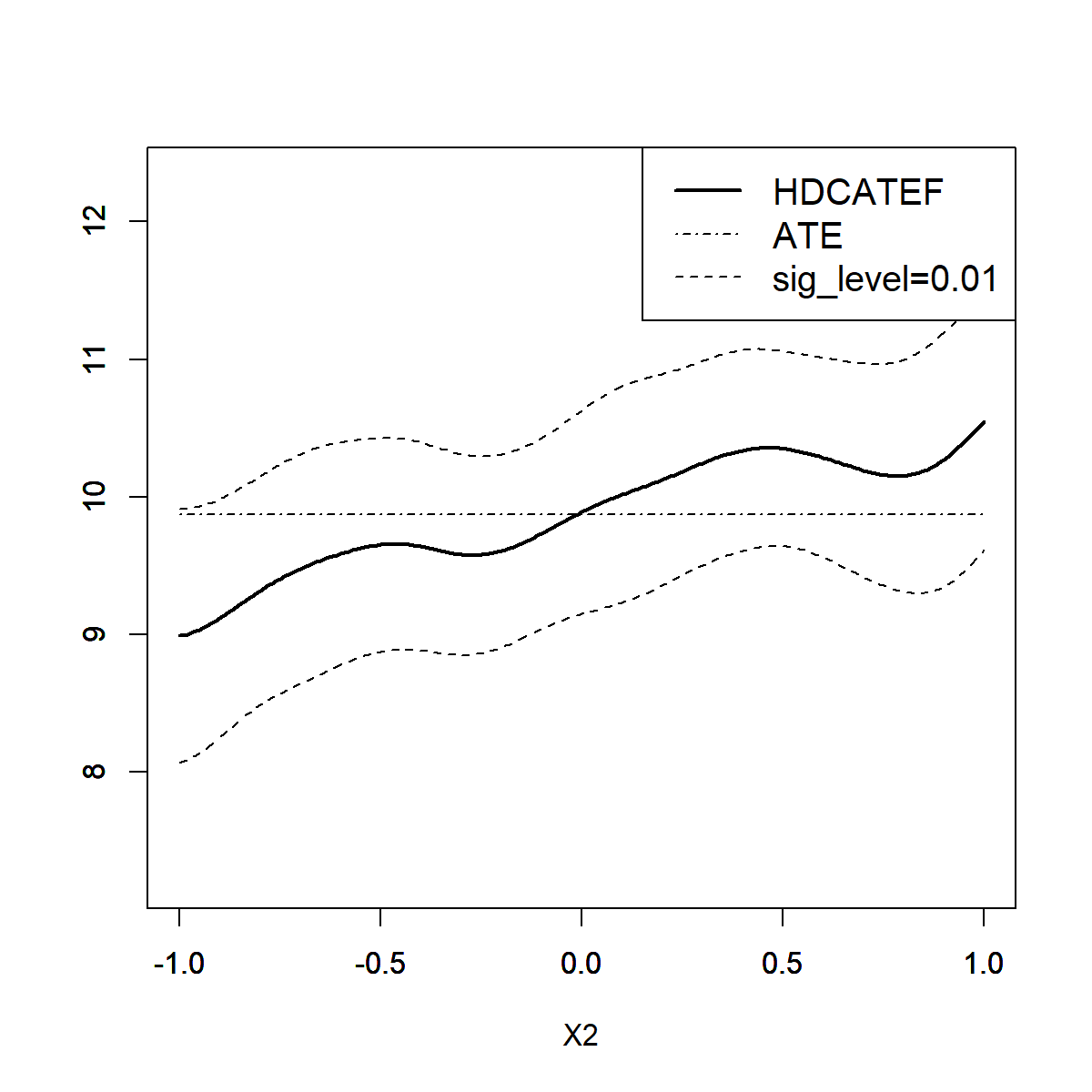HDCATE Estimation (using user-defined LASSO on the first stage) We shall see the similar result, since the user-defined method in this example is the same as the built-in method (LASSO). To clear the user-defined first-stage method and return to the default built-in method, simply call: HDCATE.unset_first_stage(model) Example 2 (Random Forest): Here we provide an additional example for another popular ML method: Random Forest, to further show how users can easily plug their own ML methods into this package: # propensity function is misspecified, for example x_formula <- "X1+X2+X3+X4+X5+X6" # create a model model <- HDCATE(data=data, y_name='Y', d_name='D', x_formula=x_formula) # =========== User-defined ML approach start =========== # using the template above, we can write: my_method_to_fit_expectation <- function(df) { # fit the input data.frame (df), where the first column is outcome value (Y) # and the rest are covariates in x_formula randomForest::randomForest(as.formula(paste0('Y', "~", x_formula)), data = df) # return a fitted object } my_method_to_predict_expectation <- function(fitted_model, df) { # use the fitted object (fitted_model) to predict the conditional expectation # fucntion on the input data.frame (df) predict(fitted_model, df) # return a vector of predicted values } my_method_to_fit_propensity <- function(df) { # fit the input data.frame (df), where the first column is dummy treatment # variable (D) and the rest are covariates in x_formula randomForest::randomForest(as.formula(paste0('D', "~", x_formula)), data = df) # return a fitted object } my_method_to_predict_propensity <- function(fitted_model, df) { # use the fitted object (fitted_model) to predict the propensity score # fucntion on the input data.frame (df) predict(fitted_model, df) # return a vector of predicted values } HDCATE.set_first_stage( model, fit.treated=my_method_to_fit_expectation, fit.untreated=my_method_to_fit_expectation, fit.propensity=my_method_to_fit_propensity, predict.treated=my_method_to_predict_expectation, predict.untreated=my_method_to_predict_expectation, predict.propensity=my_method_to_predict_propensity ) # =========== User-defined ML approach end =========== # set conditional variable in CATE, same as above HDCATE.set_condition_var(model, 'X2', min=-1, max=1, step=0.01) # fit, inference and plot HDCATE.fit(model) HDCATE.inference(model) HDCATE.plot(model)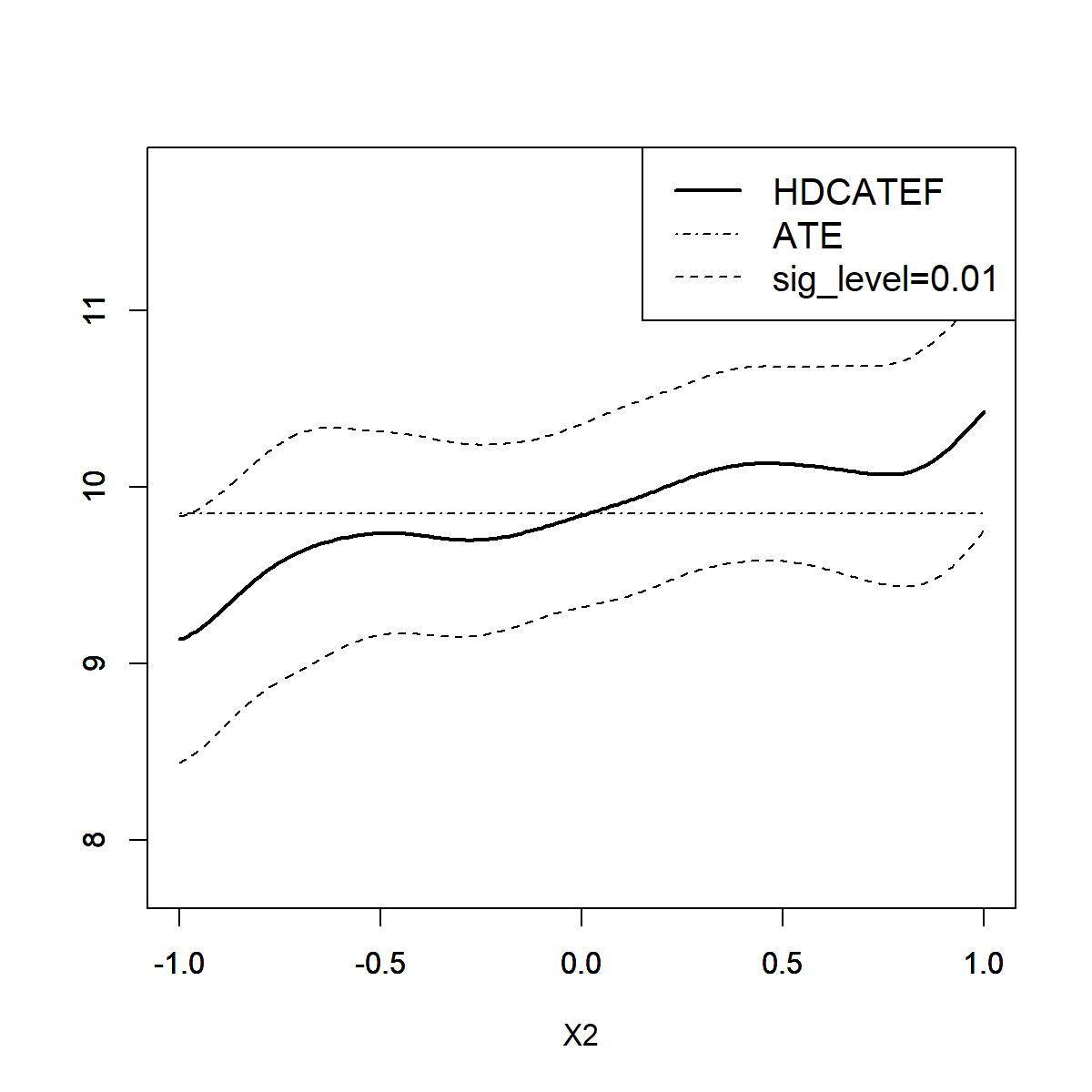HDCATE Estimation (using user-defined Random Forest model on the first stage) As shown above, after applying user-defined Random Forest approach into this package, the CATE estimation result seems even better than that using LASSO in respect of confidence bands. Futhermore, users may want to extract the fitted ML model for analysis, this can be achieved by accessing the propensity_score_model_list, untreated_cond_exp_model_list and treated_cond_exp_model_list attributes of the model object for the fitted propensity score model, fitted conditional expectation model (for untreated) and fitted conditional expectation model (for treated), respectively. The value of these attributes are “List of K”, where K is the number of folds, K=1 when using the default full-sample estimator. For example, we may want to calculate the variable importance in fitting treated conditional expectations using the fitted random forest model: # the list have only one element since we use full-sample estimator rf_model <- model$treated_cond_exp_model_list[]

# then, we can use the fitted object rf_model for futher analysis.
# the rest of codes are omitted since they are unconcerned for this package.
# ...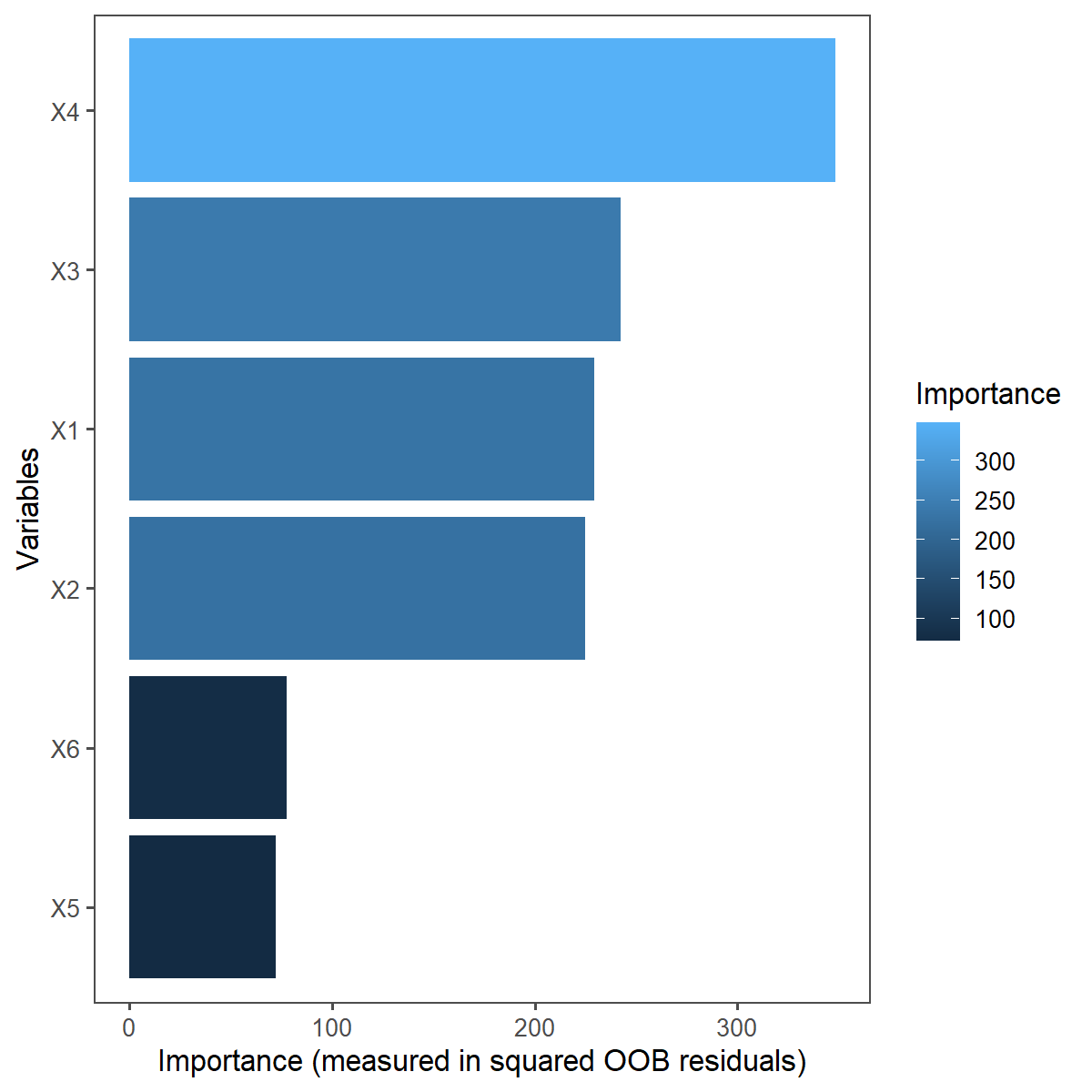Variable Importance Extracted from the User-defined Random Forest Model

We can see that in this example, the powerful random forest model captures the actual relevant variable of conditional expectation functions of potential outcome (i.e. X1, X2, X3 and X4) correctly.

Similar practice applies for other ML methods.

### User-defined Inference Options

Users can define their own inference procedure by passing the following arguments into HDCATE.inference():

• sig_level: set significant level of the confidence bands, the default is 0.01, passing a vector of significant levels is also allowed.
• n_rep_boot: set the number of bootstrap replications, the default is 1000.

For example, we may want to construct uniform confidence bands with a larger number of bootstrap replications=3000, and use another significant level=5%:

HDCATE.inference(model, sig_level=0.05, n_rep_boot=3000)

### User-defined Bandwidth

On the second stage, we use rule-of-thumb to decide the bandwidth in the local linear regression, the bandwidth can also be manually set before calling HDCATE.fit():

HDCATE.set_bw(model, 0.15)  # for example, set bandwidth=0.15

To reset to default rule-of-thumb approach, call:

HDCATE.set_bw(model)

## Other Details

Other details for using this package can be found on the detailed package documentation or the help files below:

help(HDCATE)
help(HDCATE.set_condition_var)
help(HDCATE.fit)
help(HDCATE.inference)
help(HDCATE.plot)
help(HDCATE.use_cross_fitting)
help(HDCATE.use_full_sample)
help(HDCATE.set_first_stage)
help(HDCATE.unset_first_stage)
help(HDCATE.set_bw)